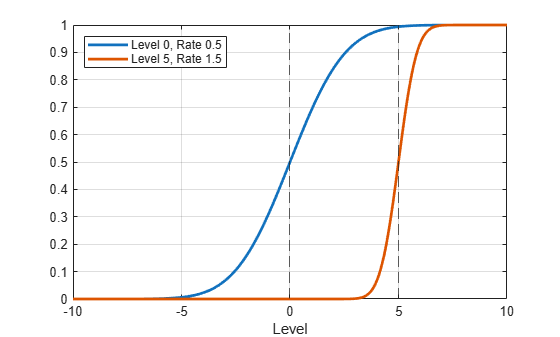# ttdata

Transition function data

Since R2021b

## Syntax

``T = ttdata(tt,z)``
``T = ttdata(tt,z,UseZeroLevels=tf)``

## Description

`ttdata` evaluates the transition function for observations of the threshold variable. To plot transition functions of threshold transitions, use `ttplot`.

example

````T = ttdata(tt,z)` returns transition function data `T` for the threshold transitions in `tt` at values of the threshold variable `z`.```

example

````T = ttdata(tt,z,UseZeroLevels=tf)` returns transformation function data with all levels set to 0 when `tf` is `true`.```

## Examples

collapse all

Create logistic threshold transitions at levels 0 and 5.

```t = [0 5]; tt = threshold(t,Type="logistic");```

`tt` is a `threshold` object. By default, the rate of each logistic transition function is `1`.

Evaluate the transition function at a sequence of transition variable data from -10 to 10.

```z = -10:0.01:10; F = ttdata(tt,z); size(F)```
```ans = 1×2 2001 2 ```

`F` is a 2001-by-2 vector of transition function data. Each column is the transition function data for the corresponding threshold in `tt.Levels`.

To facilitate comparisons among transition rates, the `Type="graph"` option of `ttplot` graphs all transition functions at the same level. This example shows how to graph transition functions each at their respective level.

Create normal threshold transitions at levels 0 and 5 with rates 0.5 and 1.5, respectively.

`tt = threshold([0 5],Type="normal",Rates=[0.5 1.5]);`

Evaluate the transition functions at their respective level (the default), and then evaluate them each relative to level 0. Specify a sequence of transition variable data from -10 to 10.

```z = -10:0.01:10; n = numel(z); T0 = ttdata(tt,z); T1 = ttdata(tt,z,UseZeroLevels=true);```

`T0` is an `n`-by-1 vector of raw transition function data evaluated at the grid of transition variable data. `T1` is an `n`-by-1 vector of transition function data translated to be centered at level 0.

Plot both sets of transition functions separately.

```% Raw transition functions figure plot(z,T0,LineWidth=2) xline(tt.Levels,'--') grid on xlabel("Level") legend(["Level 0, Rate 0.5" "Level 5, Rate 1.5"],Location="northwest")``````% Shifted transition functions figure plot(z,T1,LineWidth=2) grid on xlabel("Distance from Level") legend(["Level 0, Rate 0.5" "Level 5, Rate 1.5"],Location="northwest")```## Input Arguments

collapse all

Threshold transitions, with `NumStates` states, specified as a `threshold` object. `tt` must be fully specified (no `NaN` entries).

Threshold variable data, specified as a numeric vector.

Data Types: `double`

Flag indicating whether to compute data with all levels set to 0, specified as a value in this table:

ValueDescription
`true`Computes transformation function data with all levels set to 0. This setting is useful for comparing transition rates.
`false`Computes raw transformation function data.

Example: `UseZeroLevels=true`

Data Types: `logical`

## Output Arguments

collapse all

Transition function data F(`z`,`tt.Levels`,`tt.Rates`), returned as a numeric matrix. F is specified by `tt.TransitionFunctionData`.

Data in `T` gives transition function values relative to each level in `tt.Levels`. The number of rows of `T` is equal to the length of `z` and the number of columns is equal to the number of levels. For details, see `threshold`.

## Version History

Introduced in R2021b